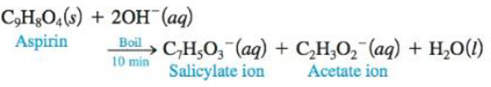# One method for determining the purity of aspirin (C 9 H 8 O 4 ) is to hydrolyze it with NaOH solution and then to titrate the remaining NaOH. The reaction of aspirin with NaOH is as follows: A sample of aspirin with a mass of 1.427 g was boiled in 50.00 mL of 0.500 M NaOH. After the solution was cooled, it took 31.92 mL of 0.289 M HCl to titrate the excess NaOH. Calculate the purity of the aspirin. What indicator should be used for this titration? Why?### Chemistry: An Atoms First Approach

2nd Edition
Steven S. Zumdahl + 1 other
Publisher: Cengage Learning
ISBN: 9781305079243

#### Solutions

Chapter
Section### Chemistry: An Atoms First Approach

2nd Edition
Steven S. Zumdahl + 1 other
Publisher: Cengage Learning
ISBN: 9781305079243
Chapter 14, Problem 96AE
Textbook Problem
24 views

## One method for determining the purity of aspirin (C9H8O4) is to hydrolyze it with NaOH solution and then to titrate the remaining NaOH. The reaction of aspirin with NaOH is as follows:A sample of aspirin with a mass of 1.427 g was boiled in 50.00 mL of 0.500 M NaOH. After the solution was cooled, it took 31.92 mL of 0.289 M HCl to titrate the excess NaOH. Calculate the purity of the aspirin. What indicator should be used for this titration? Why?

Interpretation Introduction

Interpretation: One of the methods for determining the purity of aspirin is to hydrolyze it with NaOH solution and then titrating the remaining NaOH . The reaction of aspirin with NaOH is given. The purity of the given amount of aspirin and the indicator suitable to be used is to be determined.

Concept introduction: A technique in which the concentration of an unknown solution is calculated by using a solution of a known concentration is known as titration.

A substance that depicts a change in color corresponding to a chemical change in a solution is known as an indicator.

To determine: The purity of the given amount of aspirin and the indicator suitable to be used.

### Explanation of Solution

Explanation

To find the number of moles of aspirin and  number of moles of NaOH

The given mass of aspirin is 1.427g .

The volume of NaOH is 50.0mL(0.050L) .

The volume of HCl is 31.92mL(0.03192L) .

The molarity of NaOH is 0.500M .

The molarity of HCl is 0.289M .

The molar mass of aspirin is 180g/mol .

The stated chemical reaction is,

C9H8O4(s)+2OH(aq)C7H5O3(aq)+C2H3O2(aq)+H2O(l)

The molar mass is calculated by the formula,

Numberofmoles=GivenmassMolarmass

Substitute the value of the given mass of aspirin and its molar mass in the above expression.

Numberofmoles=1.427g180g/mol=7.92×10-3mol_

The number of moles is also calculated by the formula,

Numberofmoles=Volume(L)×Molarity

Substitute the value of the volume and molarity in the above expression.

For NaOH ,

Numberofmoles=0.050L×0.500M=0.025mol_

For HCl ,

Numberofmoles=0.03192L×0.289M=9.2×10-3mol_

To find the moles of aspirin needed

The HCl reacts with the excess hydroxide present in the solution

### Still sussing out bartleby?

Check out a sample textbook solution.

See a sample solution

#### The Solution to Your Study Problems

Bartleby provides explanations to thousands of textbook problems written by our experts, many with advanced degrees!

Get Started

Find more solutions based on key concepts
A binding .site for RNA polymerase is called a ___ . a. gene b. promoter c. codon d. protein

Biology: The Unity and Diversity of Life (MindTap Course List)

Eating disorders are common only in cultures where extreme thinness is an ideal. Who in society do you think se...

Nutrition: Concepts and Controversies - Standalone book (MindTap Course List)

How could you detect Earths precession t examining star chats from ancient Egypt?

Horizons: Exploring the Universe (MindTap Course List)

An earthquake can produce a seiche in a lake in which the water sloshes back and forth from end to end with rem...

Physics for Scientists and Engineers, Technology Update (No access codes included)

Steel is heavier than water. How can a steel ship float?

Oceanography: An Invitation To Marine Science, Loose-leaf Versin